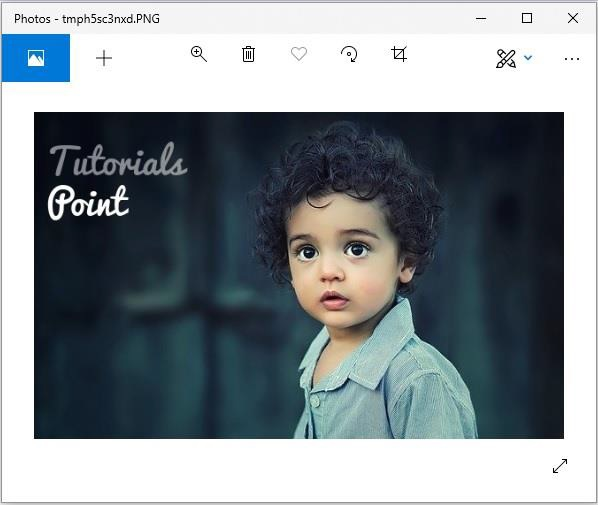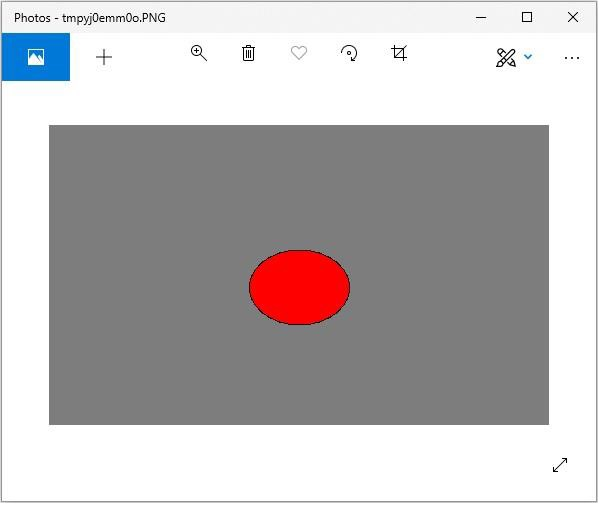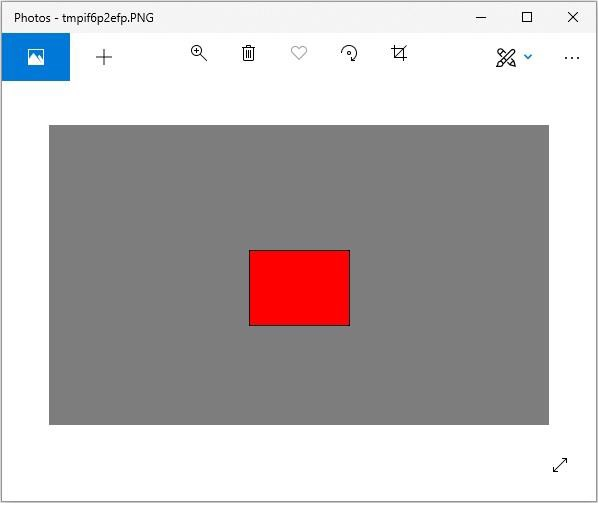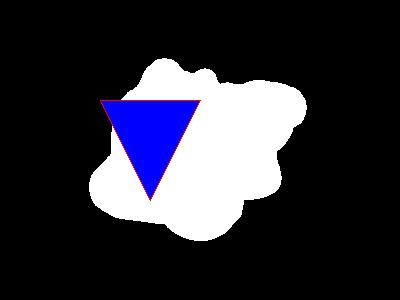# Python Pillow - ImageDraw Module

The ‘ImageDraw’ module provides simple 2D graphics support for Image Object. Generally, we use this module to create new images, annotate or retouch existing images and to generate graphics on the fly for web use.

The graphics commands support the drawing of shapes and annotation of text.

• An image can be well-thought-out to be a two-dimensional array of pixels (picture elements). A pixel is the smallest dot of color being supported.

• The origin of the two-dimensional co-ordinate system used by ImageDraw, is in the upper left corner of the image.

• The pillow color schemes we use is RGB. The color RGB representation and support is provided by the module ImageColor.

• bitmap, OpenType or TrueType are the acceptable fonts for text annotations.

• Most of the drawing commands may require a bounding box parameter that specifies the area on the image to which the command is to be applied.

• A sequence of co-ordinates can be represented as [ (x0, y0), (x1, y1),…(xn, yn)].

• For some drawing commands, we require angle values.

### Example

Following python example draws a line across the given image −

```#Import required libraries
import sys
from PIL import Image, ImageDraw

#Create Image object
im = Image.open("images/logo.jpg")

#Draw line
draw = ImageDraw.Draw(im)
draw.line((0, 0) + im.size, fill=128)
draw.line((0, im.size, im.size, 0), fill=128)

#Show image
im.show()
```

### Output

If you save the above program as Example.py and execute, it draws a line across the image and displays it using standard PNG display utility, as follows −## Canvas

• An ImageDraw is a Pillow drawable surface (i.e., a canvas) of an Image.

• ImageDraw.Draw(img) returns a drawable canvas representation of Image parameter img. The background of the canvas is the "img" image.

### Example

Following python example draws text on the given image −

```#Import required modules from Pillow package
from PIL import Image, ImageDraw, ImageFont

# get an image
base = Image.open('images/boy.jpg').convert('RGBA')

# make a blank image for the text, initialized to transparent text color
txt = Image.new('RGBA', base.size, (255,255,255,0))

# get a font
fnt = ImageFont.truetype('E:/PythonPillow/Fonts/Pacifico.ttf', 40)

# get a drawing context
d = ImageDraw.Draw(txt)

# draw text, half opacity
d.text((14,14), "Tutorials", font=fnt, fill=(255,255,255,128))

# draw text, full opacity
d.text((14,60), "Point", font=fnt, fill=(255,255,255,255))
out = Image.alpha_composite(base, txt)

#Show image
out.show()
```

### Output## Drawing Shapes using ‘ImageDraw’ module

ImageDraw module allows us to create different shapes by first creating a drawing object with the image you want to work with and then apply it. Some of the common shapes we can draw using ‘ImageDraw’ module are as follows −

### Line

Following is, the syntax to draw a line using python pillow −

```draw.line(xy, fill=None, width=0)
```

The line() method draws a line from the upper left to lower right corners of bounding box xy and canvas. The line is filled using color fill. Default values of None and 0 respectively are for the parameters fill and width which are optional.

### Example

```from PIL import Image, ImageDraw

img = Image.new('RGB', (500, 300), (125, 125, 125))
draw = ImageDraw.Draw(img)
draw.line((200, 100, 300, 200), fill=(0, 0, 0), width=10)

img.show()
```

### Output### Eclipse

Following is, the syntax to draw an ellipse using python pillow −

```draw.ellipse(xy, fill=None, outline=None)
```

The ellipse() method draws the ellipse surrounded by bounding box xy on draw. The shape is filled using color fill and the perimeter in color outline. Default values of None are for the parameters fill and width which are optional.

### Example

```from PIL import Image, ImageDraw

img = Image.new('RGB', (500, 300), (125, 125, 125))
draw = ImageDraw.Draw(img)

draw.ellipse((200, 125, 300, 200), fill=(255, 0, 0), outline=(0, 0, 0))
img.show()
```

### Output### Rectangle

Following is, the syntax to draw a rectangle using python pillow −

```draw.rectangle(xy, fill=None, outline=None)
```

The rectangle() method draws the rectangle given bounding box xy on draw. The shape is filled using color fill and the perimeter in color outline. Default values of None are for the parameters fill and width which are optional.

```from PIL import Image, ImageDraw

img = Image.new('RGB', (500, 300), (125, 125, 125))
draw = ImageDraw.Draw(img)

draw.rectangle(
(200, 125, 300, 200),
fill=(255, 0, 0),
outline=(0, 0, 0))
img.show()
```

### Output### Polygon

Following is, the syntax to draw a rectangle using python pillow −

```draw.polygon(seq, fill=None, outline=None)
```

The polygon() method draws a polygon connecting with straight lines the co-ordinate sequence locations seq on draw. The first and last co-ordinates in seq are also connected by a straight-line. The shape is filled using color fill and the perimeter in color outline. Parameters fill and outline are optional with default values None.

```from PIL import Image, ImageDraw

img = Image.new('RGB', (500, 300), (125, 125, 125))
draw = ImageDraw.Draw(img)

draw.polygon(
((200, 200), (300, 100), (250, 50)),
fill=(255, 0, 0),
outline=(0, 0, 0))
img.show()
```

### Output## Useful Video Courses

Video

#### Python Online Training

187 Lectures 17.5 hours

Video

#### Python Essentials Online Training

55 Lectures 8 hours

Video

#### Learn Python Programming in 100 Easy Steps

136 Lectures 11 hours

Video

#### Python with Data Science

Best Seller

75 Lectures 13 hours

Video

#### Python 3 from scratch to become a developer in demand

Best Seller

70 Lectures 8.5 hours

Video

#### Python Data Science basics with Numpy, Pandas and Matplotlib

Most Popular

63 Lectures 6 hours